### OpenCV 3.x with Python By Example - Second Edition4.1 (7 reviews total)
By Gabriel Garrido , Prateek Joshi

• Constantly updated with 100+ new titles each month
• Breadth and depth in over 1,000+ technologies

Computer vision is found everywhere in modern technology. OpenCV for Python enables us to run computer vision algorithms in real time. With the advent of powerful machines, we have more processing power to work with. Using this technology, we can seamlessly integrate our computer vision applications into the cloud. Focusing on OpenCV 3.x and Python 3.6, this book will walk you through all the building blocks needed to build amazing computer vision applications with ease.

We start off by manipulating images using simple filtering and geometric transformations. We then discuss affine and projective transformations and see how we can use them to apply cool advanced manipulations to your photos like resizing them while keeping the content intact or smoothly removing undesired elements. We will then cover techniques of object tracking, body part recognition, and object recognition using advanced techniques of machine learning such as artificial neural network. 3D reconstruction and augmented reality techniques are also included. The book covers popular OpenCV libraries with the help of examples.

This book is a practical tutorial that covers various examples at different levels, teaching you about the different functions of OpenCV and their actual implementation. By the end of this book, you will have acquired the skills to use OpenCV and Python to develop real-world computer vision applications.

Publication date:
January 2018
Publisher
Packt
Pages
268
ISBN
9781788396905

## Chapter 1. Applying Geometric Transformations to Images

In this chapter, we are going to learn how to apply cool geometric effects to images. Before we get started, we need to install OpenCV-Python. We will explain how to compile and install the necessary libraries to follow every example in this book.

By the end of this chapter, you will know:

• How to install OpenCV-Python
• How to read, display, and save images
• How to convert to multiple color spaces
• How to apply geometric transformations such as translation, rotation, and scaling
• How to use affine and projective transformations to apply funny geometric effects to photos

## Installing OpenCV-Python

In this section, we explain how to install OpenCV 3.X with Python 2.7 on multiple platforms. If you desire it, OpenCV 3.X also supports the use of Python 3.X and it will be fully compatible with the examples in this book. Linux is recommended as the examples in this book were tested on that OS.

### Windows

In order to get OpenCV-Python up and running, we need to perform the following steps:

1. Install Python: Make sure you have Python 2.7.x installed on your machine. If you don't have it, you can install it from: https://www.python.org/downloads/windows/.
2. Install NumPy: NumPy is a great package to do numerical computing in Python. It is very powerful and has a wide variety of functions. OpenCV-Python plays nicely with NumPy, and we will be using this package a lot during the course of this book. You can install the latest version from: http://sourceforge.net/projects/numpy/files/NumPy/.

We need to install all these packages in their default locations. Once we install Python and NumPy, we need to ensure that they're working fine. Open up the Python shell and type the following:

` >>> import numpy `

If the installation has gone well, this shouldn't throw up any errors. Once you confirm it, you can go ahead and download the latest OpenCV version from: http://opencv.org/downloads.html.

Once you finish downloading it, double-click to install it. We need to make a couple of changes, as follows:

1. Navigate to `opencv/build/python/2.7/`.
2. You will see a file named `cv2.pyd`. Copy this file to `C:/Python27/lib/site-packages`.

You're all set! Let's make sure that OpenCV is working. Open up the Python shell and type the following:

` >>> import cv2`

If you don't see any errors, then you are good to go! You are now ready to use OpenCV-Python.

### macOS X

To install OpenCV-Python, we will be using Homebrew. Homebrew is a great package manager for macOS X and it will come in handy when you are installing various libraries and utilities on macOS X. If you don't have Homebrew, you can install it by running the following command in your terminal:

```\$ ruby -e "\$(curl -fsSL
https://raw.githubusercontent.com/Homebrew/install/master/install)"
```

Even though OS X comes with inbuilt Python, we need to install Python using Homebrew to make our lives easier. This version is called brewed Python. Once you install Homebrew, the next step is to install brewed Python. Open up the terminal, and type the following:

`\$ brew install python`

This will automatically install it as well. Pip is a package management tool to install packages in Python, and we will be using it to install other packages. Let's make sure the brewed Python is working correctly. Go to your terminal and type the following:

`\$ which python`

You should see `/usr/local/bin/python` printed on the terminal. This means that we are using the brewed Python, and not the inbuilt system Python. Now that we have installed brewed Python, we can go ahead and add the repository, `homebrew/science`, which is where OpenCV is located. Open the terminal and run the following command:

`\$ brew tap homebrew/science`

Make sure the NumPy package is installed. If not, run the following in your terminal:

```\$ pip install numpy
```

Now, we are ready to install OpenCV. Go ahead and run the following command from your terminal:

```\$ brew install opencv --with-tbb --with-opengl
```

OpenCV is now installed on your machine, and you can find it at `/usr/local/Cellar/opencv/3.1.0/`. You can't use it just yet. We need to tell Python where to find our OpenCV packages. Let's go ahead and do that by symlinking the OpenCV files. Run the following commands from your terminal (please, double check that you are actually using the right versions, as they might be slightly different):

```\$ cd /Library/Python/2.7/site-packages/
\$ ln -s /usr/local/Cellar/opencv/3.1.0/lib/python2.7/site-packages/cv.py
cv.py
\$ ln -s /usr/local/Cellar/opencv/3.1.0/lib/python2.7/site-packages/cv2.so
cv2.so
```

You're all set! Let's see if it's installed properly. Open up the Python shell and type the following:

`> import cv2`

If the installation went well, you will not see any error messages. You are now ready to use OpenCV in Python.

If you want to use OpenCV within a virtual environment, you could follow the instructions in the Virtual environments section, applying small changes to each of the commands for macOS X.

### Linux (for Ubuntu)

First, we need to install the OS requirements:

```[compiler] \$ sudo apt-get install build-essential
[required] \$ sudo apt-get install cmake git libgtk2.0-dev pkg-config
libavcodec-dev libavformat-dev libswscale-dev git
libgstreamer0.10-dev libv4l-dev
[optional] \$ sudo apt-get install python-dev python-numpy libtbb2
libtbb-dev libjpeg-dev libpng-dev libtiff-dev libjasper-dev
libdc1394-22-dev
```

Once the OS requirements are installed, we need to download and compile the latest version of OpenCV along with several supported flags to let us implement the following code samples. Here we are going to install Version 3.3.0:

```\$ mkdir ~/opencv
\$ git clone -b 3.3.0 https://github.com/opencv/opencv.git opencv
\$ cd opencv
\$ git clone https://github.com/opencv/opencv_contrib.git opencv_contrib
\$ mkdir release
\$ cd release
\$ cmake -D CMAKE_BUILD_TYPE=RELEASE -D CMAKE_INSTALL_PREFIX=/usr/local  -D INSTALL_PYTHON_EXAMPLES=ON   -D INSTALL_C_EXAMPLES=OFF -D OPENCV_EXTRA_MODULES_PATH=~/opencv/opencv_contrib/modules -D BUILD_PYTHON_SUPPORT=ON -D WITH_XINE=ON -D WITH_OPENGL=ON -D WITH_TBB=ON -D WITH_EIGEN=ON -D BUILD_EXAMPLES=ON -D BUILD_NEW_PYTHON_SUPPORT=ON -D WITH_V4L=ON -D BUILD_EXAMPLES=ON ../
\$ make -j4 ; echo 'Running in 4 jobs'
\$ sudo make install```

If you are using Python 3, place `-D` + flags together, as you see in the following command:

`cmake -DCMAKE_BUILD_TYPE=RELEASE....`

#### Virtual environments

If you are using virtual environments to keep your test environment completely separate from the rest of your OS, you could install a tool called virtualenvwrapper by following this tutorial: https://virtualenvwrapper.readthedocs.io/en/latest/.

To get OpenCV running on this virtualenv, we need to install the NumPy package:

`\$(virtual_env) pip install numpy`

Following all the previous steps, just add the following three flags on compilation by `cmake` (pay attention that flag `CMAKE_INSTALL_PREFIX` is being redefined):

```\$(<env_name>) > cmake ...
-D CMAKE_INSTALL_PREFIX=~/.virtualenvs/<env_name> \
-D PYTHON_EXECUTABLE=~/.virtualenvs/<env_name>/bin/python
-D PYTHON_PACKAGES_PATH=~/.virtualenvs/<env_name>/lib/python<version>/site-packages ...```

Let's make sure that it's installed correctly. Open up the Python shell and type the following:

`> import cv2`

If you don't see any errors, you are good to go.

##### Troubleshooting

If the `cv2` library was not found, identify where the library was compiled. It should be located at `/usr/local/lib/python2.7/site-packages/cv2.so`. If that is the case, make sure your Python version matches the one package that has been stored, otherwise just move it into the according `site-packages` folder of Python, including same for virtualenvs.

During `cmake` command execution, try to join `-DMAKE`... and the rest of the `-D` lines. Moreover, if execution fails during the compiling process, some libraries might be missing from the OS initial requirements. Make sure you installed them all.

You can find an official tutorial about how to install the latest version of OpenCV on Linux at the following website: http://docs.opencv.org/trunk/d7/d9f/tutorial_linux_install.html.

If you are trying to compile using Python 3, and `cv2.so` is not installed, make sure you installed OS dependency Python 3 and NumPy.

#### OpenCV documentation

OpenCV official documentation is at http://docs.opencv.org/. There are three documentation categories: Doxygen, Sphinx, and Javadoc.

In order to obtain a better understanding of how to use each of the functions used during this book, we encourage you to open one of those doc pages and research the different uses of each OpenCV library method used in our examples. As a suggestion, Doxygen documentation has more accurate and extended information about the use of OpenCV.

## Reading, displaying, and saving images

Let's see how we can load an image in OpenCV-Python. Create a file named `first_program.py` and open it in your favorite code editor. Create a folder named `images` in the current folder, and make sure that you have an image named `input.jpg` in that folder.

Once you do that, add the following lines to that Python file:

```import cv2
```cv2.imshow('Input image', img)
cv2.waitKey()```

If you run the preceding program, you will see an image being displayed in a new window.

### What just happened?

Let's understand the previous piece of code, line by line. In the first line, we are importing the OpenCV library. We need this for all the functions we will be using in the code. In the second line, we are reading the image and storing it in a variable. OpenCV uses NumPy data structures to store the images. You can learn more about NumPy at `http://www.numpy.org`.

So if you open up the Python shell and type the following, you will see the datatype printed on the terminal:

```> import cv2
> type(img)
<type 'numpy.ndarray'>```

In the next line, we display the image in a new window. The first argument in `cv2.imshow` is the name of the window. The second argument is the image you want to display.

You must be wondering why we have the last line here. The function, `cv2.waitKey()`, is used in OpenCV for keyboard binding. It takes a number as an argument, and that number indicates the time in milliseconds. Basically, we use this function to wait for a specified duration, until we encounter a keyboard event. The program stops at this point, and waits for you to press any key to continue. If we don't pass any argument, or if we pass as the argument, this function will wait for a keyboard event indefinitely.

The last statement, `cv2.waitKey(n)`, performs the rendering of the image loaded in the step before. It takes a number that indicates the time in milliseconds of rendering. Basically, we use this function to wait for a specified duration until we encounter a keyboard event. The program stops at this point, and waits for you to press any key to continue. If we don't pass any argument, or if we pass 0 as the argument, this function waits for a keyboard event indefinitely.

OpenCV provides multiple ways of loading an image. Let's say we want to load a color image in grayscale mode, we can do that using the following piece of code:

```import cv2
cv2.imshow('Grayscale', gray_img)
cv2.waitKey()```

Here, we are using the ImreadFlag, as `cv2.IMREAD_GRAYSCALE`, and loading the image in grayscale mode, although you may find more read modes in the official documentation.

You can see the image displayed in the new window. Here is the input image: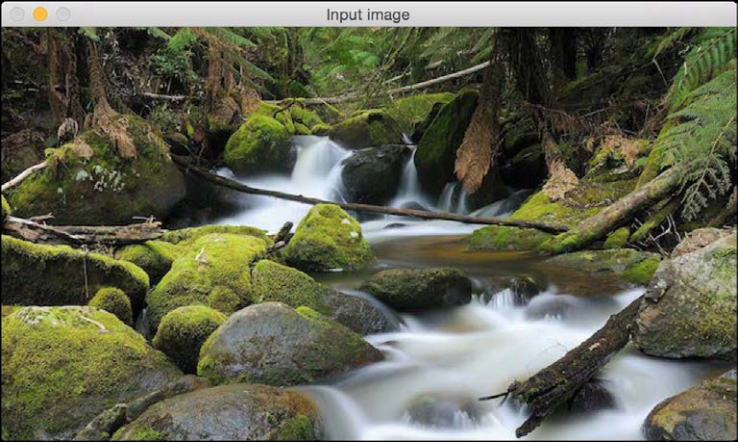Following is the corresponding grayscale image: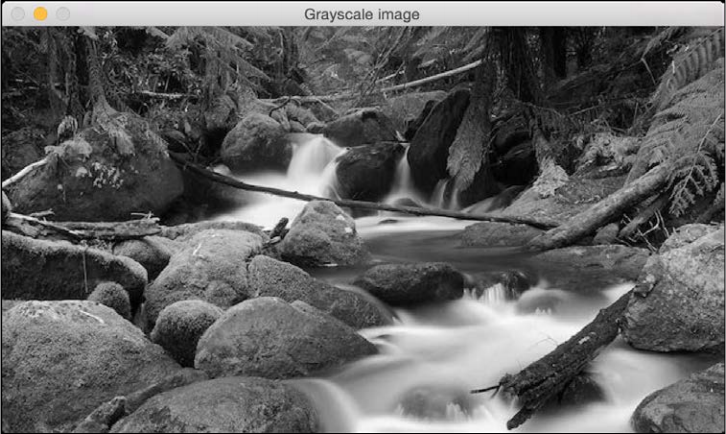We can save this image as a file as well:

`cv2.imwrite('images/output.jpg', gray_img)`

This will save the grayscale image as an output file named `output.jpg`. Make sure you get comfortable with reading, displaying, and saving images in OpenCV, because we will be doing this quite a bit during the course of this book.

### Changing image format

We can save this image as a file as well, and change the original image format to PNG:

```import cv2
cv2.imwrite('images/output.png', img, [cv2.IMWRITE_PNG_COMPRESSION])```

The `imwrite()` method will save the grayscale image as an output file named `output.png`. This is done using PNG compression with the help of ImwriteFlag and `cv2.IMWRITE_PNG_COMPRESSION`. The ImwriteFlag allows the output image to change the format, or even the image quality.

## Image color spaces

In computer vision and image processing, color space refers to a specific way of organizing colors. A color space is actually a combination of two things, a color model and a mapping function. The reason we want color models is because it helps us in representing pixel values using tuples. The mapping function maps the color model to the set of all possible colors that can be represented.

There are many different color spaces that are useful. Some of the more popular color spaces are RGB, YUV, HSV, Lab, and so on. Different color spaces provide different advantages. We just need to pick the color space that's right for the given problem. Let's take a couple of color spaces and see what information they provide:

• RGB: Probably the most popular color space. It stands for Red, Green, and Blue. In this color space, each color is represented as a weighted combination of red, green, and blue. So every pixel value is represented as a tuple of three numbers corresponding to red, green, and blue. Each value ranges between 0 and 255.
• YUV: Even though RGB is good for many purposes, it tends to be very limited for many real-life applications. People started thinking about different methods to separate the intensity information from the color information. Hence, they came up with the YUV color space. Y refers to the luminance or intensity, and U/V channels represent color information. This works well in many applications because the human visual system perceives intensity information very differently from color information.
• HSV: As it turned out, even YUV was still not good enough for some applications. So people started thinking about how humans perceive color, and they came up with the HSV color space. HSV stands for Hue, Saturation, and Value. This is a cylindrical system where we separate three of the most primary properties of colors and represent them using different channels. This is closely related to how the human visual system understands color. This gives us a lot of flexibility as to how we can handle images.

### Converting color spaces

Considering all the color spaces, there are around 190 conversion options available in OpenCV. If you want to see a list of all available flags, go to the Python shell and type the following:

```import cv2
print([x for x in dir(cv2) if x.startswith('COLOR_')])```

You will see a list of options available in OpenCV for converting from one color space to another. We can pretty much convert any color space to any other color space. Let's see how we can convert a color image to a grayscale image:

```import cv2
gray_img = cv2.cvtColor(img, cv2.COLOR_RGB2GRAY)
cv2.imshow('Grayscale image', gray_img)
cv2.waitKey()```

#### What just happened?

We use the `cvtColor` function to convert color spaces. The first argument is the input image and the second argument specifies the color space conversion.

### Splitting image channels

You can convert to YUV by using the following flag:

`yuv_img = cv2.cvtColor(img, cv2.COLOR_BGR2YUV)`

The image will look something like the following one: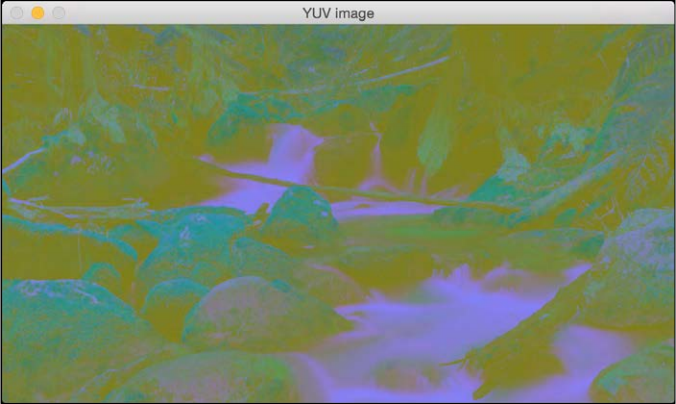This may look like a deteriorated version of the original image, but it's not. Let's separate out the three channels:

```# Alternative 1
y,u,v = cv2.split(yuv_img)
cv2.imshow('Y channel', y)
cv2.imshow('U channel', u)
cv2.imshow('V channel', v)
cv2.waitKey()```
```# Alternative 2 (Faster)
cv2.imshow('Y channel', yuv_img[:, :, 0])
cv2.imshow('U channel', yuv_img[:, :, 1])
cv2.imshow('V channel', yuv_img[:, :, 2])
cv2.waitKey()```

Since `yuv_img` is a NumPy (which provides dimensional selection operators), we can separate out the three channels by slicing it. If you look at `yuv_img.shape`, you will see that it is a 3D array. So once you run the preceding piece of code, you will see three different images. Following is the `Y``channel`: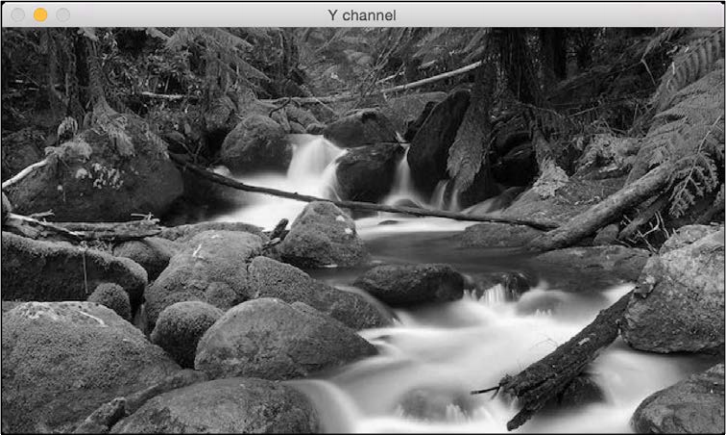The channel is basically the grayscale image. Next is the `U channel`: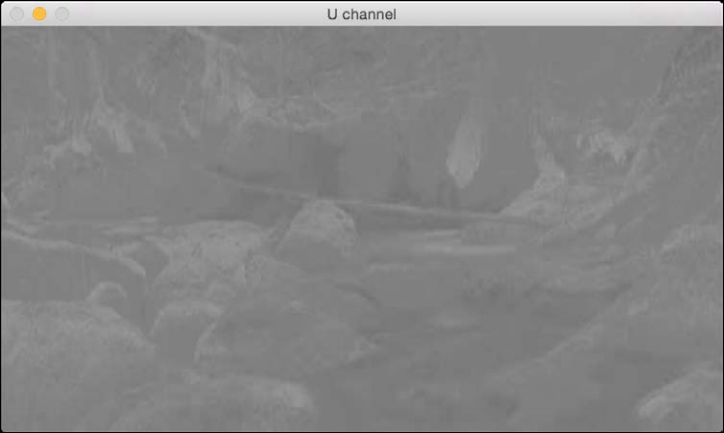And lastly, the `V channel`: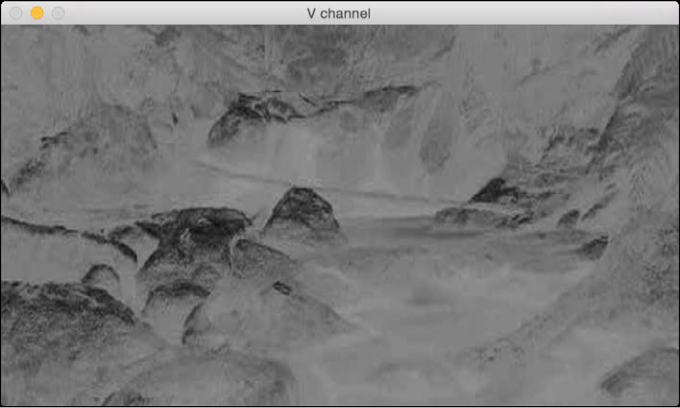As we can see here, the channel is the same as the grayscale image. It represents the intensity values, and channels represent the color information.

### Merging image channels

Now we are going to read an image, split it into separate channels, and merge them to see how different effects can be obtained out of different combinations:

```img = cv2.imread('./images/input.jpg', cv2.IMREAD_COLOR)
g,b,r = cv2.split(img)
gbr_img = cv2.merge((g,b,r))
rbr_img = cv2.merge((r,b,r))
cv2.imshow('Original', img)
cv2.imshow('GRB', gbr_img)
cv2.imshow('RBR', rbr_img)
cv2.waitKey()```

Here we can see how channels can be recombined to obtain different color intensities: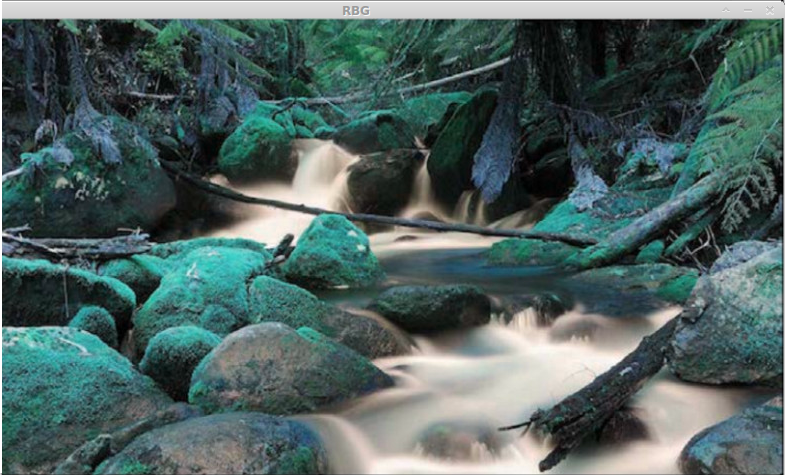In this one, the red channel is used twice so the reds are more intense: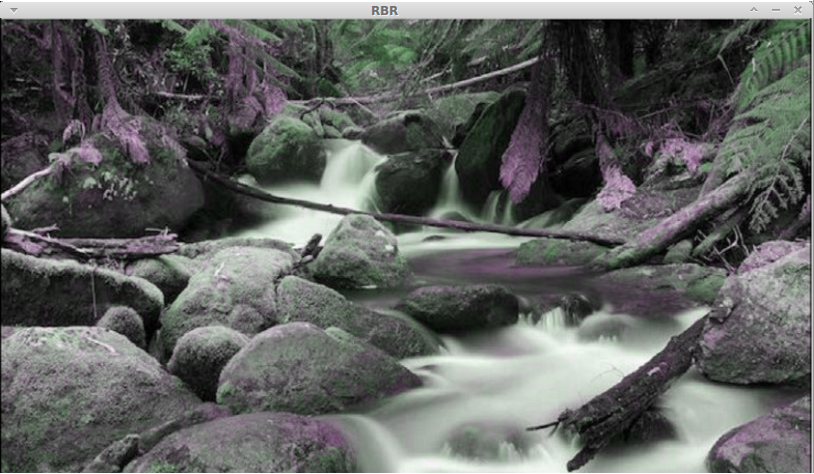This should give you a basic idea of how to convert between color spaces. You can play around with more color spaces to see what the images look like. We will discuss the relevant color spaces as and when we encounter them during subsequent chapters.

## Image translation

In this section, we will discuss shifting an image. Let's say we want to move the image within our frame of reference. In computer vision terminology, this is referred to as translation. Let's go ahead and see how we can do that:

```import cv2
import numpy as np
num_rows, num_cols = img.shape[:2]
translation_matrix = np.float32([ [1,0,70], [0,1,110] ])
img_translation = cv2.warpAffine(img, translation_matrix, (num_cols, num_rows), cv2.INTER_LINEAR)
cv2.imshow('Translation', img_translation)
cv2.waitKey()```

If you run the preceding code, you will see something like the following: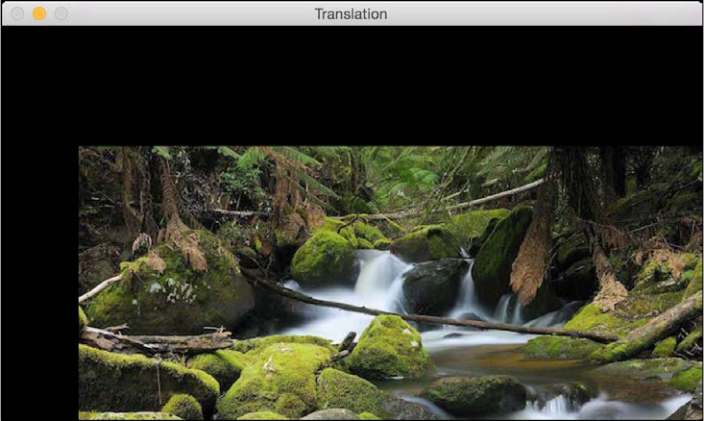### What just happened?

To understand the preceding code, we need to understand how warping works. Translation basically means that we are shifting the image by adding/subtracting the x and y coordinates. In order to do this, we need to create a transformation matrix, as follows: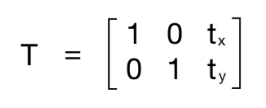Here, the tx and ty values are the x and y translation values; that is, the image will be moved by x units to the right, and by y units downwards. So once we create a matrix like this, we can use the function, `warpAffine`, to apply it to our image. The third argument in `warpAffine` refers to the number of rows and columns in the resulting image. As follows, it passes `InterpolationFlags` which defines combination of interpolation methods.

Since the number of rows and columns is the same as the original image, the resultant image is going to get cropped. The reason for this is we didn't have enough space in the output when we applied the translation matrix. To avoid cropping, we can do something like this:

```img_translation = cv2.warpAffine(img, translation_matrix,
(num_cols + 70, num_rows + 110))```

If you replace the corresponding line in our program with the preceding line, you will see the following image: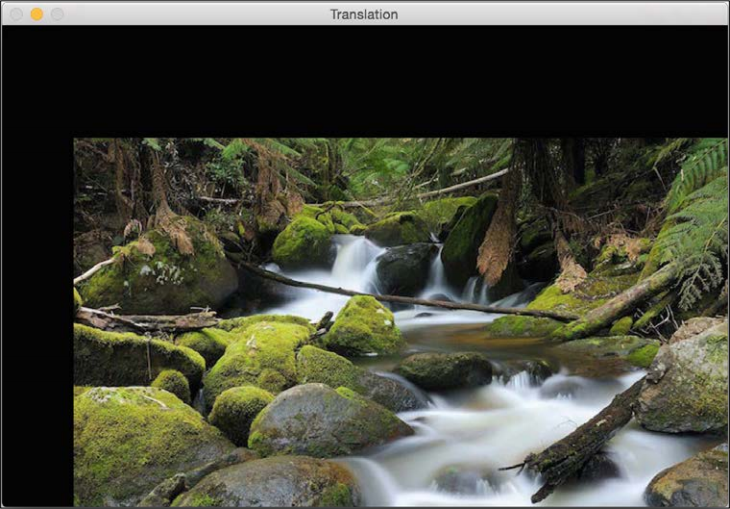Let's say you want to move the image to the middle of a bigger image frame; we can do something like this by carrying out the following:

```import cv2
import numpy as np
num_rows, num_cols = img.shape[:2]
translation_matrix = np.float32([ [1,0,70], [0,1,110] ])
img_translation = cv2.warpAffine(img, translation_matrix, (num_cols + 70, num_rows + 110))
translation_matrix = np.float32([ [1,0,-30], [0,1,-50] ])
img_translation = cv2.warpAffine(img_translation, translation_matrix, (num_cols + 70 + 30, num_rows + 110 + 50))
cv2.imshow('Translation', img_translation)
cv2.waitKey()```

If you run the preceding code, you will see an image like the following: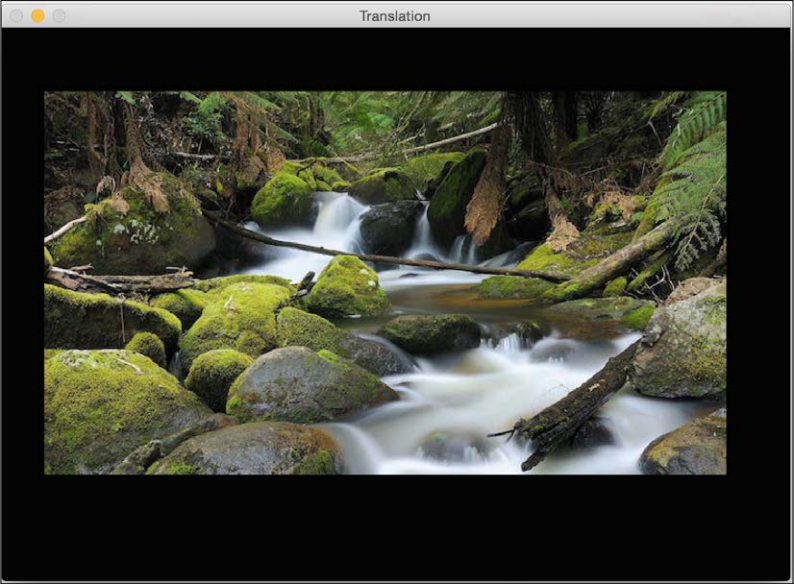Moreover, there are two more arguments, `borderMode` and `borderValue`, that allow you to fill up the empty borders of the translation with a pixel extrapolation method:

```import cv2
import numpy as np
num_rows, num_cols = img.shape[:2]
translation_matrix = np.float32([ [1,0,70], [0,1,110] ])
img_translation = cv2.warpAffine(img, translation_matrix, (num_cols, num_rows), cv2.INTER_LINEAR, cv2.BORDER_WRAP, 1)
cv2.imshow('Translation', img_translation)
cv2.waitKey()```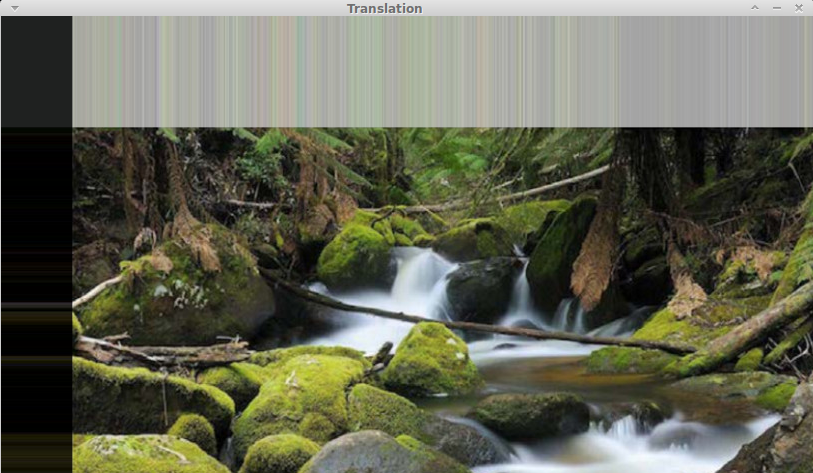## Image rotation

In this section, we will see how to rotate a given image by a certain angle. We can do it using the following piece of code:

```import cv2
import numpy as np
img = cv2.imread('images/input.jpg')num_rows, num_cols = img.shape[:2]
rotation_matrix = cv2.getRotationMatrix2D((num_cols/2, num_rows/2), 30, 0.7)
img_rotation = cv2.warpAffine(img, rotation_matrix, (num_cols, num_rows))
cv2.imshow('Rotation', img_rotation)
cv2.waitKey()```

If you run the preceding code, you will see an image like this: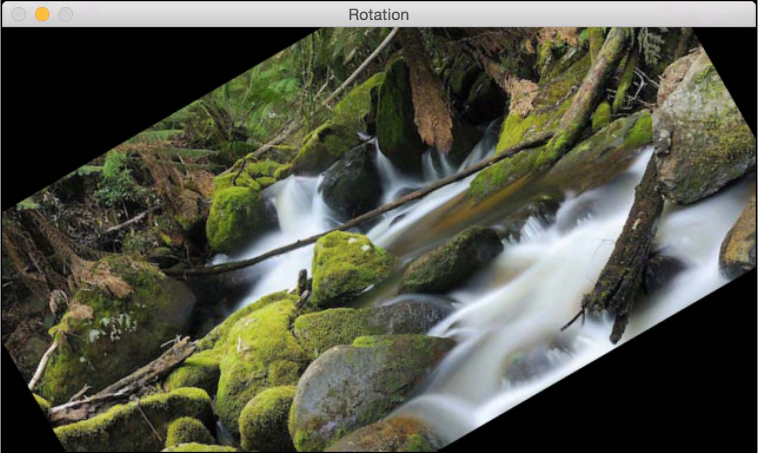### What just happened?

Using `getRotationMatrix2D`, we can specify the center point around which the image would be rotated as the first argument, then the angle of rotation in degrees, and a scaling factor for the image at the end. We use 0.7 to shrink the image by 30% so it fits in the frame.

In order to understand this, let's see how we handle rotation mathematically. Rotation is also a form of transformation, and we can achieve it by using the following transformation matrix: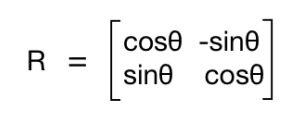Here, θ is the angle of rotation in the counterclockwise direction. OpenCV provides finer control over the creation of this matrix through the `getRotationMatrix2D` function. We can specify the point around which the image would be rotated, the angle of rotation in degrees, and a scaling factor for the image. Once we have the transformation matrix, we can use the warpAffine function to apply this matrix to any image.

As we can see from the previous figure, the image content goes out of bounds and gets cropped. In order to prevent this, we need to provide enough space in the output image.

Let's go ahead and do that using the translation functionality we discussed earlier:

```import cv2
import numpy as np

num_rows, num_cols = img.shape[:2]

translation_matrix = np.float32([ [1,0,int(0.5*num_cols)], [0,1,int(0.5*num_rows)] ])
rotation_matrix = cv2.getRotationMatrix2D((num_cols, num_rows), 30, 1)

img_translation = cv2.warpAffine(img, translation_matrix, (2*num_cols, 2*num_rows))
img_rotation = cv2.warpAffine(img_translation, rotation_matrix, (num_cols*2, num_rows*2))

cv2.imshow('Rotation', img_rotation)
cv2.waitKey()```

If we run the preceding code, we will see something like this: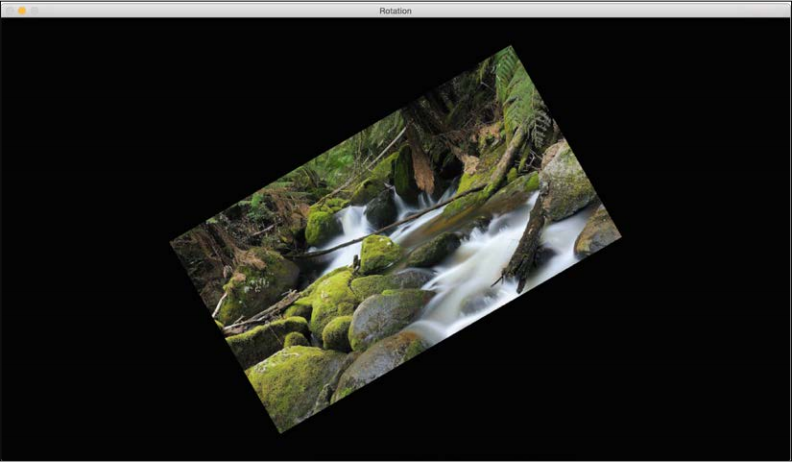## Image scaling

In this section, we will discuss resizing an image. This is one of the most common operations in computer vision. We can resize an image using a scaling factor, or we can resize it to a particular size. Let's see how to do that:

```import cv2
img_scaled = cv2.resize(img,None,fx=1.2, fy=1.2, interpolation = cv2.INTER_LINEAR)
cv2.imshow('Scaling - Linear Interpolation', img_scaled)
img_scaled = cv2.resize(img,None,fx=1.2, fy=1.2, interpolation = cv2.INTER_CUBIC)
cv2.imshow('Scaling - Cubic Interpolation', img_scaled)
img_scaled = cv2.resize(img,(450, 400), interpolation = cv2.INTER_AREA)
cv2.imshow('Scaling - Skewed Size', img_scaled)
cv2.waitKey()```

### What just happened?

Whenever we resize an image, there are multiple ways to fill in the pixel values. When we are enlarging an image, we need to fill up the pixel values in between pixel locations. When we are shrinking an image, we need to take the best representative value. When we are scaling by a non-integer value, we need to interpolate values appropriately, so that the quality of the image is maintained. There are multiple ways to do interpolation. If we are enlarging an image, it's preferable to use linear or cubic interpolation. If we are shrinking an image, it's preferable to use area-based interpolation. Cubic interpolation is computationally more complex, and hence slower than linear interpolation. However, the quality of the resulting image will be higher.

OpenCV provides a function called resize to achieve image scaling. If you don't specify a size (by using `None`), then it expects the x and y scaling factors. In our example, the image will be enlarged by a factor of 1.2. If we do the same enlargement using cubic interpolation, we can see that the quality improves, as seen in the following figure. The following screenshot shows what linear interpolation looks like:Here is the corresponding cubic interpolation: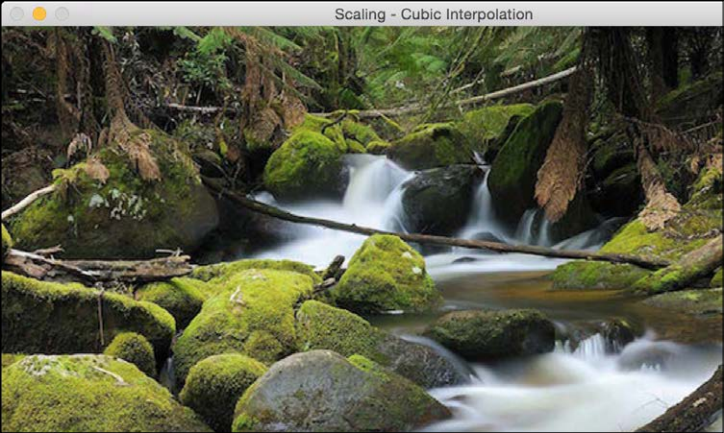If we want to resize it to a particular size, we can use the format shown in the last resize instance. We can basically skew the image and resize it to whatever size we want. The output will look something like the following: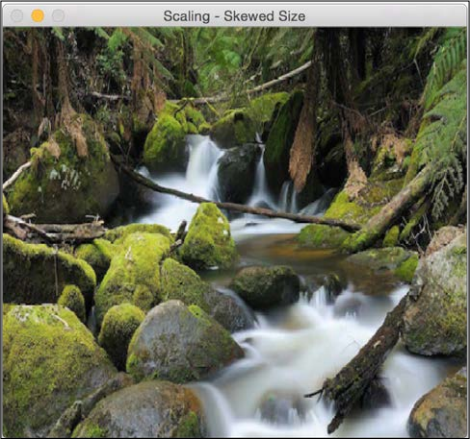## Affine transformations

In this section, we will discuss the various generalized geometrical transformations of 2D images. We have been using the function `warpAffine` quite a bit over the last couple of sections, it's about time we understood what's happening underneath.

Before talking about affine transformations, let's learn what Euclidean transformations are. Euclidean transformations are a type of geometric transformation that preserve length and angle measures. If we take a geometric shape and apply Euclidean transformation to it, the shape will remain unchanged. It might look rotated, shifted, and so on, but the basic structure will not change. So technically, lines will remain lines, planes will remain planes, squares will remain squares, and circles will remain circles.

Coming back to affine transformations, we can say that they are generalizations of Euclidean transformations. Under the realm of affine transformations, lines will remain lines, but squares might become rectangles or parallelograms. Basically, affine transformations don't preserve lengths and angles.

In order to build a general affine transformation matrix, we need to define the control points. Once we have these control points, we need to decide where we want them to be mapped. In this particular situation, all we need are three points in the source image, and three points in the output image. Let's see how we can convert an image into a parallelogram-like image:

```import cv2
import numpy as np
rows, cols = img.shape[:2]
src_points = np.float32([[0,0], [cols-1,0], [0,rows-1]])
dst_points = np.float32([[0,0], [int(0.6*(cols-1)),0], [int(0.4*(cols-1)),rows-1]])
affine_matrix = cv2.getAffineTransform(src_points, dst_points)
img_output = cv2.warpAffine(img, affine_matrix, (cols,rows))
cv2.imshow('Input', img)
cv2.imshow('Output', img_output)
cv2.waitKey()```

### What just happened?

As we discussed earlier, we are defining control points. We just need three points to get the affine transformation matrix. We want the three points in `src_points` to be mapped to the corresponding points in `dst_points`. We are mapping the points as shown in the following image: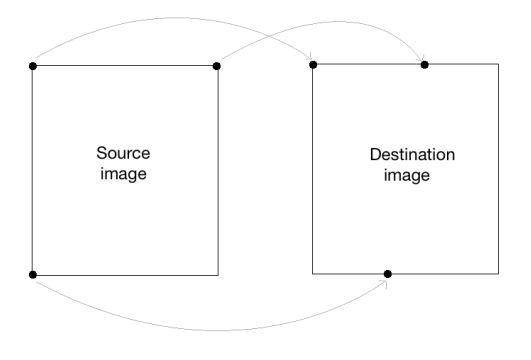To get the transformation matrix, we have a function called in OpenCV. Once we have the affine transformation matrix, we use the function to apply this matrix to the input image.

Following is the input image: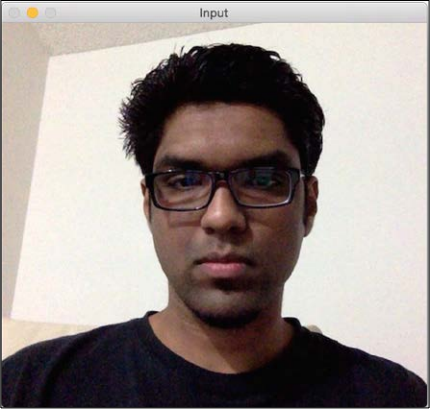If you run the preceding code, the output will look something like this: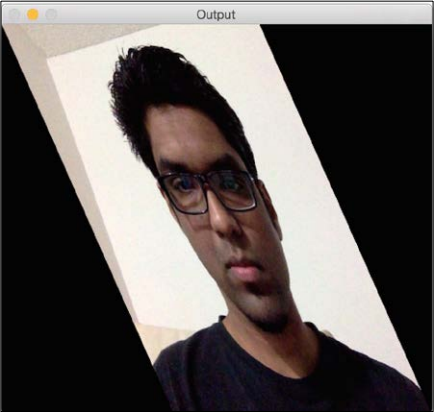We can also get the mirror image of the input image. We just need to change the control points in the following way:

```src_points = np.float32([[0,0], [cols-1,0], [0,rows-1]])
dst_points = np.float32([[cols-1,0], [0,0], [cols-1,rows-1]])```

Here, the mapping looks something like this: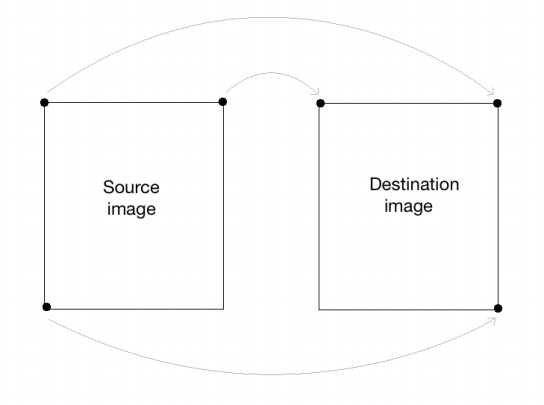If you replace the corresponding lines in our affine transformation code with these two lines, you will get the following result: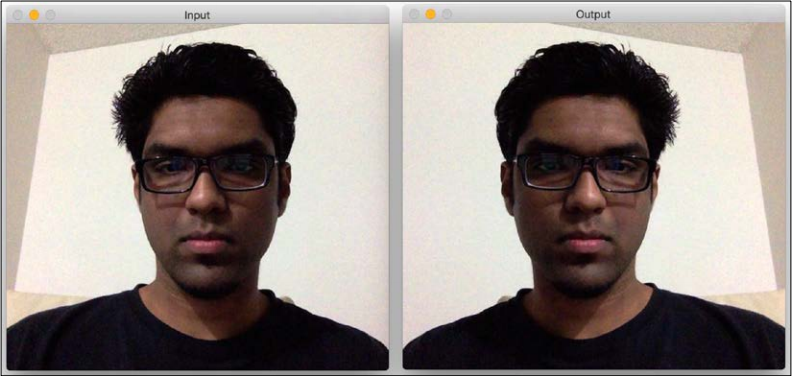## Projective transformations

Affine transformations are nice, but they impose certain restrictions. A projective transformation, on the other hand, gives us more freedom. In order to understand projective transformations, we need to understand how projective geometry works. We basically describe what happens to an image when the point of view is changed. For example, if you are standing right in front of a sheet of paper with a square drawn on it, it will look like a square.

Now, if you start tilting that sheet of paper, the square will start looking more and more like a trapezoid. Projective transformations allow us to capture this dynamic in a nice mathematical way. These transformations preserve neither sizes nor angles, but they do preserve incidence and cross-ratio.

### Note

You can read more about incidence and cross-ratio at http://en.wikipedia.org/wiki/Incidence_(geometry) and http://en.wikipedia.org/wiki/Cross-ratio.

Now that we know what projective transformations are, let's see if we can extract more information here. We can say that any two images on a given plane are related by a homography. As long as they are in the same plane, we can transform anything into anything else. This has many practical applications such as augmented reality, image rectification, image registration, or the computation of camera motion between two images. Once the camera rotation and translation have been extracted from an estimated homography matrix, this information may be used for navigation, or to insert models of 3D objects into an image or video. This way, they are rendered with the correct perspective, and it will look like they were part of the original scene.

Let's go ahead and see how to do this:

```import cv2
import numpy as np
rows, cols = img.shape[:2]
src_points = np.float32([[0,0], [cols-1,0], [0,rows-1], [cols-1,rows-1]])
dst_points = np.float32([[0,0], [cols-1,0], [int(0.33*cols),rows-1], [int(0.66*cols),rows-1]])
projective_matrix = cv2.getPerspectiveTransform(src_points, dst_points)
img_output = cv2.warpPerspective(img, projective_matrix, (cols,rows))
cv2.imshow('Input', img)
cv2.imshow('Output', img_output)
cv2.waitKey()```

If you run the preceding code you will see the funny-looking output, such as the following screenshot: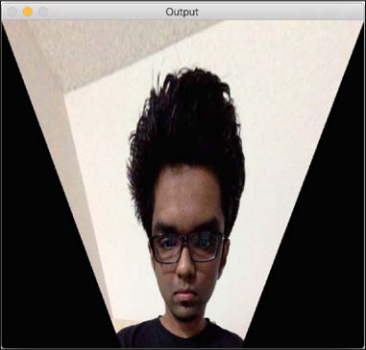### What just happened?

We can choose four control points in the source image and map them to the destination image. Parallel lines will not remain parallel lines after the transformation. We use a function called `getPerspectiveTransform` to get the transformation matrix.

Let's apply a couple of fun effects using projective transformation, and see what they look like. All we need to do is change the control points to get different effects.

Here's an example: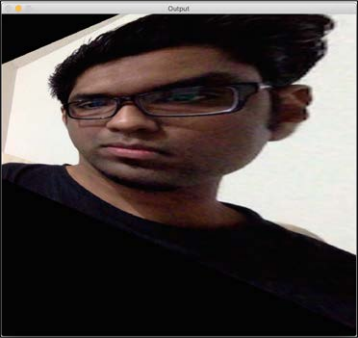The control points are as follows:

```src_points = np.float32([[0,0], [0,rows-1], [cols/2,0],[cols/2,rows-1]])
dst_points = np.float32([[0,100], [0,rows-101], [cols/2,0],[cols/2,rows-1]])```

As an exercise, you should map the preceding points on a plane, and see how the points are mapped (just like we did earlier, while discussing affine transformations). You will get a good understanding about the mapping system, and you can create your own control points. If we want to obtain the same effect on the y axis we could apply the previous transformation.

## Image warping

Let's have some more fun with the images and see what else we can achieve. Projective transformations are pretty flexible, but they still impose some restrictions on how we can transform the points. What if we want to do something completely random? We need more control, right? It just so happens we can do that as well. We just need to create our own mapping, and it's not that difficult. Following are a few effects that you can achieve with image warping:

>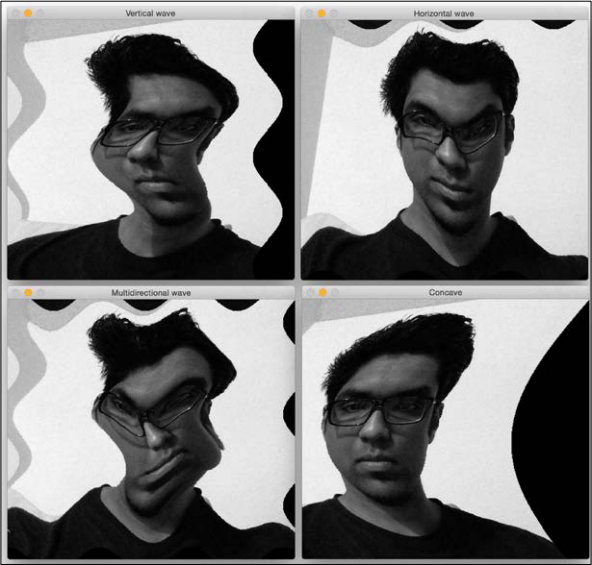Here is the code to create these effects:

```import cv2
import numpy as np
import math

rows, cols = img.shape

#####################
# Vertical wave

img_output = np.zeros(img.shape, dtype=img.dtype)

for i in range(rows):
for j in range(cols):
offset_x = int(25.0 * math.sin(2 * 3.14 * i / 180))
offset_y = 0
if j+offset_x < rows:
img_output[i,j] = img[i,(j+offset_x)%cols]
else:
img_output[i,j] = 0

cv2.imshow('Input', img)
cv2.imshow('Vertical wave', img_output)

#####################
# Horizontal wave

img_output = np.zeros(img.shape, dtype=img.dtype)

for i in range(rows):
for j in range(cols):
offset_x = 0
offset_y = int(16.0 * math.sin(2 * 3.14 * j / 150))
if i+offset_y < rows:
img_output[i,j] = img[(i+offset_y)%rows,j]
else:
img_output[i,j] = 0

cv2.imshow('Horizontal wave', img_output)

#####################
# Both horizontal and vertical

img_output = np.zeros(img.shape, dtype=img.dtype)

for i in range(rows):
for j in range(cols):
offset_x = int(20.0 * math.sin(2 * 3.14 * i / 150))
offset_y = int(20.0 * math.cos(2 * 3.14 * j / 150))
if i+offset_y < rows and j+offset_x < cols:
img_output[i,j] = img[(i+offset_y)%rows,(j+offset_x)%cols]
else:
img_output[i,j] = 0

cv2.imshow('Multidirectional wave', img_output)

#####################
# Concave effect

img_output = np.zeros(img.shape, dtype=img.dtype)

for i in range(rows):
for j in range(cols):
offset_x = int(128.0 * math.sin(2 * 3.14 * i / (2*cols)))
offset_y = 0
if j+offset_x < cols:
img_output[i,j] = img[i,(j+offset_x)%cols]
else:
img_output[i,j] = 0

cv2.imshow('Concave', img_output)

cv2.waitKey() ```

## Summary

In this chapter, we learned how to install OpenCV-Python on various platforms. We discussed how to read, display, and save images. We talked about the importance of various color spaces, and how we can convert to multiple color spaces, splitting and merging them. We learned how to apply geometric transformations to images, and understood how to use those transformations to achieve cool geometric effects. We discussed the underlying formulation of transformation matrices, and how we can formulate different kinds of transformations based on our needs. We learned how to select control points based on the required geometric transformation. We discussed projective transformations and learned how to use image warping to achieve any given geometric effect.

In the next chapter, we are going to discuss edge detection and image filtering. We can apply a lot of visual effects using image filters, and the underlying formation gives us a lot of freedom to manipulate images in creative ways.

• ##### Gabriel Garrido

Gabriel Garrido is a multifaceted and versatile software engineer with more than 7 years of experience in developing web applications for companies such as Telefonica, Trivago, and Base7Booking. He has a degree in computer science from the University of Granada, Spain.

He is passionate about coding, focusing on its quality and spending hours working on personal projects based on technologies such as computer vision, artificial intelligence, and augmented reality. Taking part in hackathons is one of his hobbies. He has won a couple of prizes for implementing beta software for a Google Cardboard hackathon and another for a travel assistant at a TNOOZ hackathon.

Browse publications by this author
• ##### Prateek Joshi

Prateek Joshi is an artificial intelligence researcher, an author of several books, and a TEDx speaker. He has been featured in Forbes 30 Under 30, CNBC, TechCrunch, Silicon Valley Business Journal, and many more publications. He is the founder of Pluto AI, a venturefunded Silicon Valley start-up building an intelligence platform for water facilities. He graduated from the University of Southern California with a Master's degree specializing in Artificial Intelligence. He has previously worked at NVIDIA and Microsoft Research.

Browse publications by this author

### Latest Reviews

(7 reviews total)